# 位运算（3）-- 高级运用

## 一、二进制中1的个数

int oneCount(unsigned int n){
int c = 0;
while(n){
c += n & 1;
n >>= 1;
}
return c;
}

int oneCount1(unsigned int n){
int c = 0;
while(n){
n &= (n - 1);   // 清除最低位的1
c++;
}
return c;
}

int oneCount2(unsigned int n){

/*获得偶数位的值*/   /*获得奇数位的值*/        // 常数的二进制表示
n = (n & 0x55555555) + ((n >> 1)  & 0x55555555);    // 0101010101010101...
n = (n & 0x33333333) + ((n >> 2)  & 0x33333333);    // 0011001100110011...
n = (n & 0x0f0f0f0f) + ((n >> 4)  & 0x0f0f0f0f);    // 0000111100001111...
n = (n & 0x00ff00ff) + ((n >> 8)  & 0x00ff00ff);    // 0000000011111111...
n = (n & 0x0000ffff) + ((n >> 16) & 0x0000ffff);    // 0000000000000000...

return n;
}

int oneCount3(unsigned int n){
return __builtin_popcount(n);   // VS: __popcnt(n)
}

## 二、最左侧1问题

way 1.：通过逐次移位获得最高位（左移右移皆可），复杂度较高。

int left_most_one_1(int n){
int pos = -1;
while(n){
n >>= 1;
pos++;
}
return pos;
}

way 2.：通过二分法寻找最左侧1，复杂度较way1降低很多。

int left_most_one_2(int n){
if(n == 0) return -1;

int exp = 4;
int pos = (1 << exp);   // 从16的位置开始二分
while(exp > 0){
exp--;
if(n >> pos) pos += (1 << exp);
else pos -= (1 << exp);
}
return n >> pos != 0 ? pos : pos - 1;
}

way 3.：原问题等价于将整数转化成科学记数法的幂。例如，01101000 = 1.101 * 2 ^ 6。首先将整数强转为浮点数(必须用double，防止精度损失)，此时浮点数中就包含了指数部分（想想计算机是怎么表示浮点数的），将其解析出来即是最左侧1。解析方法是地址强转：获得浮点数的地址，将地址强转成int型地址，然后移位即可获得指数。

int left_most_one_3(int n){
if(!n) return -1;

double b = n;
/* 右移52位获得双精度浮点数的指数部分，再与上2047(0b11111111111)只获得指数部分，
*  最后减去偏移量(移码)，就是最终的指数部分的十进制值
*/
return ((*(long long*)&b) >> 52 & 2047) - 1023;
}

way 4.：编译器内置位运算，GCC下是__builtin_clz，VS下是__lzcnt

int left_most_one_4(int n){
return 31 - __builtin_clz(n);   // VS: __lzcnt
}

## 三、Single number

#### I

int singleNumberI(int* A, int len){
int res = 0;
for(int i = 0; i < len; ++i){
res ^= A[i];
}
return res;
}

#### II

int singleNumberII(int* A, int len){
int count, res;
memset(count, 0, sizeof(count));

for(int i = 0; i < len; ++i){
for(int j = 0; j < 32; ++j){
count[j] += A[i] >> j & 1;
}
}

for(int i = 0; i < 32; ++i){
res |= count[i] % 3 << i;   // 模3再放置到对应的位
}

return res;
}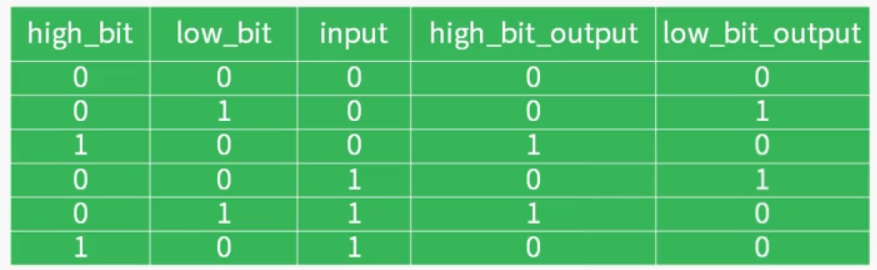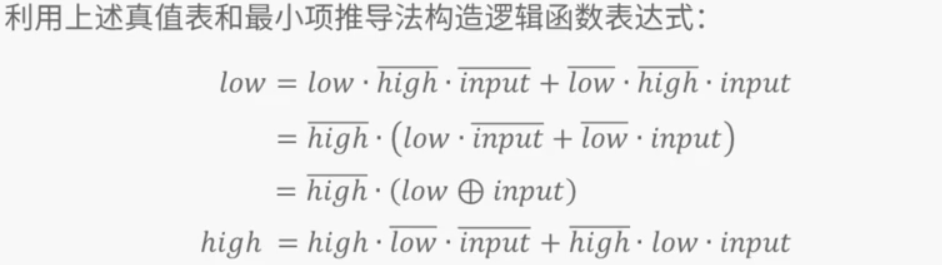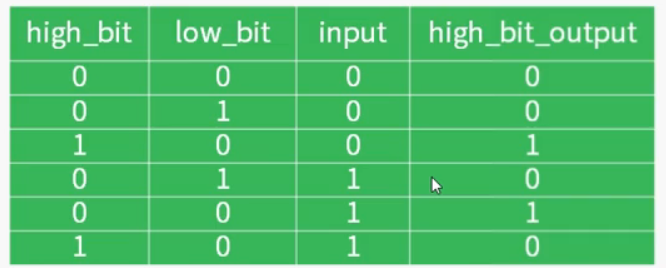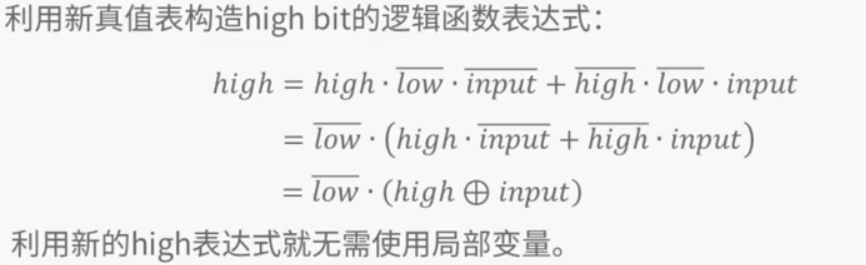int singleNumberII2(int* A, int len){
int ones = 0, twos = 0;
for(int i = 0; i < len; ++i){
ones = (ones ^ A[i]) & ~twos;
twos = (twos ^ A[i]) & ~ones;
}
return ones;
}

#### III

int* singleNumberIII(int* nums, int numSize, int* returnSize){
int* res = (int*)malloc(sizeof(int) * 2);
memset(res, 0, sizeof(int) * 2);
int xorsum = 0;

for(int i = 0; i < numSize; ++i){
xorsum ^= nums[i];
}

int low_one = xorsum & -xorsum;     // 获得最低有效位，即最低位1的位置

for(int i = 0; i < numSize; ++i){
/* 分成两类 */
if(low_one & nums[i]){
res = res ^ nums[i];
}
else{
res = res ^ nums[i];
}
}

*returnSize = 2;

return res;
}

## 四、N皇后问题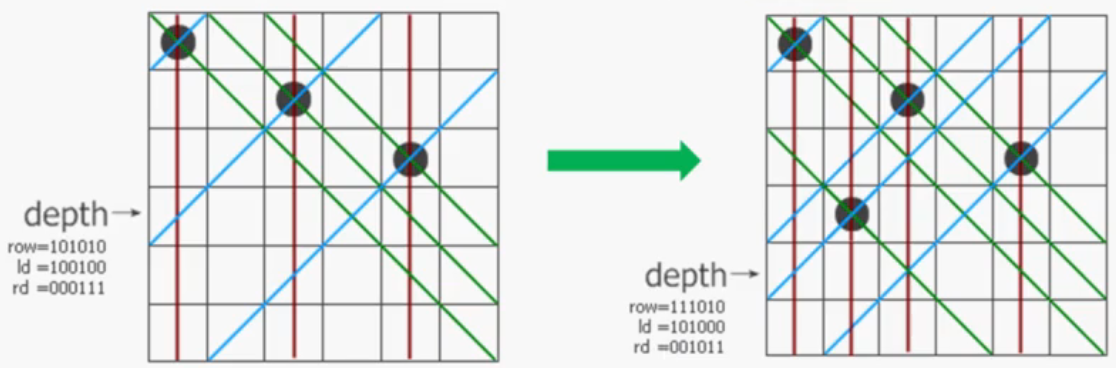const int QUEEN_NUM = 14;       // 皇后的个数
int position[QUEEN_NUM];       // 每个皇后在每一行的位置
static int all_queen = (1 << QUEEN_NUM) -1;
static int sum = 0;            // 放法

// 假设在前 t - 1 行没有冲突的情况下，第t行放置的位置是否冲突
bool check(int t){
for(int i = 0; i < t; ++i){
// 行 - 行 == 列 - 列 或者 在同一列
if(abs(t - i) == abs(position[t] - position[i]) || position[i] == position[t])
return false;
}
return true;
}

// 回溯法
void n_queen_backtrace(int row){    // 在第row行放置一个皇后
if(row == QUEEN_NUM){
sum++;
return;
}

for(int i = 0; i < QUEEN_NUM; ++i){
position[row] = i;
if(check(row)) n_queen_backtrace(row + 1);
}
}

/*
* 采用位运算的解法
* row, ld和rd, 分别表示在纵列和两个对角线方向的限制条件下该行的哪些地方不能放
*/
void n_queen_bit(int row, int ld, int rd){
if(row == all_queen){
sum++;
return;
}
// pos 表示当前行可以放置皇后的位置
int pos = all_queen & ~(row | ld | rd);
// 挑出这些位置
while(pos){
int p = pos & -pos;
pos -= p;
n_queen_bit(row + p, (ld + p) << 1, (rd + p) >> 1);
}
}

int main(){

n_queen_backtrace(0);
//n_queen_bit(0, 0, 0);
printf("%d\n", sum);

return 0;
}

## 五、格雷码

unsigned int binary2gray(unsigned int n){
return n ^ (n >> 1);
}

unsigned int gray2binary(unsigned int g){
unsigned int b = g;
while(g >>= 1){
b ^= g;
}
return b;
}

void print_binary_format(int g){
int bit = {0};

int i = 31;
while(g){
bit[i--] = g % 2;
g /= 2;
}
for(int j = 0; j < 32; ++j){
printf("%d", bit[j]);
}
}
void gen_gray_matrix(int m, int n){
for(int i = 0; i < (1 << m); ++i){      // 行
int high = (i ^ (i >> 1)) << n; // 高位部分格雷码
for(int j = 0; j < (1 << n); ++j){  // 列
int low = j ^ (j >> 1);     // 低位部分格雷码
print_binary_format(high | low);
printf(" ");
}
printf("\n");
}
}